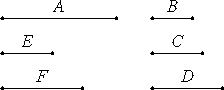# Proposition 14

If a number is the least that is measured by prime numbers, then it is not measured by any other prime number except those originally measuring it.

Let the number A be the least that is measured by the prime numbers B, C, and D.

I say that A is not measured by any other prime number except B, C, or D.

If possible, let it be measured by the prime number E, and let E not be the same as any one of the numbers B, C, or D.Now, since E measures A, let it measure it according to F, therefore E multiplied by F makes A. And A is measured by the prime numbers B, C, and D.

VII.30

But, if two numbers multiplied by one another make some number, and any prime number measures the product, then it also measures one of the original numbers, therefore each of B, C, and D measures one of the numbers E or F.

Now they do not measure E, for E is prime and not the same with any one of the numbers B, C, or D. Therefore they measure F, which is less than A, which is impossible, for A is by hypothesis the least number measured by B, C, and D.

Therefore no prime number measures A except B, C, and D.

Therefore, if a number is the least that is measured by prime numbers, then it is not measured by any other prime number except those originally measuring it.

Q.E.D.

## Guide

This proposition states that the least common multiple of a set of prime numbers is not divisible by any other prime. The least common multiple is actually the product of those primes, but that isn’t mentioned.

The proof is clear, and it depends on VII.30, that if a prime divides a product, then it divides one of the factors.